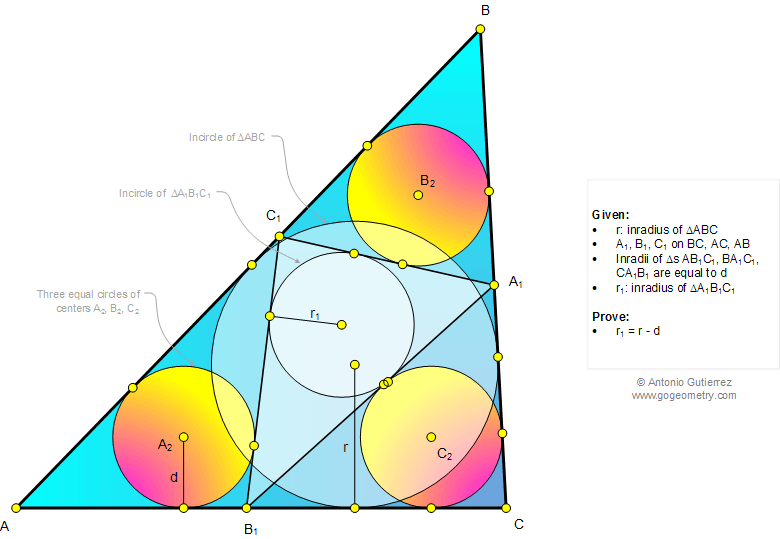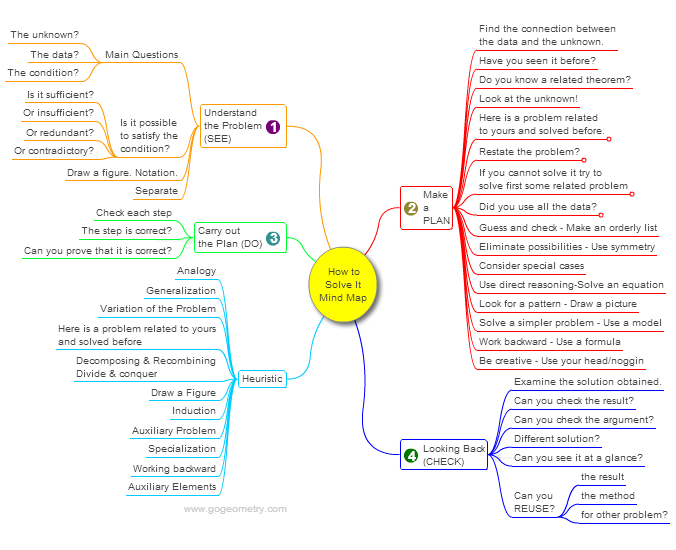# Online Math: Geometry Problem 1037: Triangle, Three equal Incircles, Tangent lines, Inradius, Length. Level: School, College.

< PREVIOUS PROBLEM  |  NEXT PROBLEM >

 The figure below shows a triangle ABC where A1, B1, and C1 are on BC, AC, and AB, respectively, so that the inradii of triangles AB1C1, BA1C1, and CA1B1 are equal to d. If r is the inradius of triangle ABC and r1 is the inradius of triangle A1B1C1, prove that r1 = r - d.## Mind Map: George Pólya's 1945 book, "How to Solve It"

George Pólya's 1945 book "How to Solve It, A new aspect of Mathematical Method", is a book describing methods of problem solving. It suggests the following steps when solving a mathematical problem: (1) First, you have to understand the problem. (2) After understanding, then make a plan. (3) Carry out the plan. (4) Look back on your work. How could it be better?
A mind map is a diagram used to visually organize information, knowledge, concepts or ideas.Home | SearchGeometry | Problems | All Problems | Open Problems | Visual Index | 10 Problems | Problems Art GalleryArt | 1031-1040 | Triangle | Circle | Incircle | Areas | Triangle area | Perimeter | Tangent Line | Email | by Antonio Gutierrez

Add a solution to the problem 1037
Last updated: Sep 19, 2014